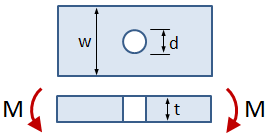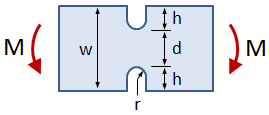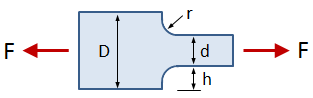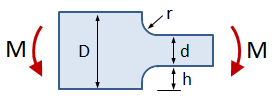﻿ Stress Concentration Calculator | MechaniCalc

This page provides a set of interactive plots for common stress concentration factors. See the reference section for more details.

### Rectangular Bar With Central Hole, Axial Force

This tab defines the stress concentration factor for a bar with a rectangular cross section and a central circular hole. The bar has an applied axial force (tensile or compressive).• w = bar width
• d = hole diameter
• t = bar thickness
• F = applied force (tensile or compressive)
Cannot display plot -- browser is out of date.

The maximum stress is calculated as σmax = Kt σnom, where Kt is the stress concentration factor as determined from the plot below, and σnom is calculated as:

Cannot display plot -- browser is out of date.

Input Geometry:

Results:

d/w:

Kt:

The value of the stress concentration factor is calculated by:

Sources:

### Rectangular Bar With Central Hole, Out-of-Plane Bending

This tab defines the stress concentration factor for a bar with a rectangular cross section and a central circular hole. The bar has an applied out-of-plane bending moment.• w = bar width
• d = hole diameter
• t = bar thickness
• M = applied bending moment
Cannot display plot -- browser is out of date.

The maximum stress is calculated as σmax = Kt σnom, where Kt is the stress concentration factor as determined from the plot below, and σnom is calculated as:

Cannot display plot -- browser is out of date.

Input Geometry:

Results:

d/t:

d/w:

Kt:

The value of the stress concentration factor is calculated by:

Sources:

### Rectangular Bar With U-Notches, Axial Force

This tab defines the stress concentration factor for a bar with a rectangular cross section and two U-notches. The bar has an applied axial force (tensile or compressive).• w = bar width
• h = notch height
• t = bar thickness
• F = applied force (tensile or compressive)
Cannot display plot -- browser is out of date.

The maximum stress is calculated as σmax = Kt σnom, where Kt is the stress concentration factor as determined from the plot below, and σnom is calculated as:

Cannot display plot -- browser is out of date.

Input Geometry:

Results:

d:

w/d:

r/d:

h/r:

Kt:

The value of the stress concentration factor is calculated by:

where the coefficients in the equation above are calculated from "Peterson's Stress Concentration Factors":

0.1 ≤ h/r ≤ 2.0 2.0 ≤ h/r ≤ 50.0
C1 0.955 + 2.169 √h/r − 0.081 (h/r) 1.037 + 1.991 √h/r + 0.002 (h/r)
C2 −1.557 − 4.046 √h/r + 1.032 (h/r) −1.886 − 2.181 √h/r − 0.048 (h/r)
C3 4.013 + 0.424 √h/r − 0.748 (h/r) 0.649 + 1.086 √h/r + 0.142 (h/r)
C4 −2.461 + 1.538 √h/r − 0.236 (h/r) 1.218 − 0.922 √h/r − 0.086 (h/r)

Sources:

### Rectangular Bar With U-Notches, Bending

This tab defines the stress concentration factor for a bar with a rectangular cross section and two U-notches. The bar has an applied in-plane bending moment.• w = bar width
• h = notch height
• t = bar thickness
• M = applied bending moment
Cannot display plot -- browser is out of date.

The maximum stress is calculated as σmax = Kt σnom, where Kt is the stress concentration factor as determined from the plot below, and σnom is calculated as:

Cannot display plot -- browser is out of date.

Input Geometry:

Results:

d:

w/d:

r/d:

h/r:

Kt:

The value of the stress concentration factor is calculated by:

where the coefficients in the equation above are calculated from "Peterson's Stress Concentration Factors":

0.1 ≤ h/r ≤ 2.0 2.0 ≤ h/r ≤ 50.0
C1 1.024 + 2.092 √h/r − 0.051 (h/r) 1.113 + 1.957 √h/r
C2 −0.630 − 7.194 √h/r + 1.288 (h/r) −2.579 − 4.017 √h/r − 0.013 (h/r)
C3 2.117 + 8.574 √h/r − 2.160 (h/r) 4.100 + 3.922 √h/r + 0.083 (h/r)
C4 −1.420 − 3.494 √h/r + 0.932 (h/r) −1.528 − 1.893 √h/r − 0.066 (h/r)

Sources:

### Rectangular Bar With Fillet, Axial Force

This tab defines the stress concentration factor for a bar with a rectangular cross section and a fillet. The bar has an applied axial force (tensile or compressive).• D = width of larger section
• d = width of smaller section
• r = radius of fillet
• t = bar thickness
• F = applied force (tensile or compressive)
Cannot display plot -- browser is out of date.

The maximum stress is calculated as σmax = Kt σnom, where Kt is the stress concentration factor as determined from the plot below, and σnom is calculated as:

Cannot display plot -- browser is out of date.

Input Geometry:

Results:

h:

r/d:

D/d:

h/r:

Kt:

The value of the stress concentration factor is calculated by:

where the coefficients in the equation above are calculated from "Roark's Formulas for Stress and Strain":

0.1 ≤ h/r ≤ 2.0 2.0 ≤ h/r ≤ 20.0
C1 1.007 + 1.000 √h/r − 0.031 (h/r) 1.042 + 0.982 √h/r − 0.036 (h/r)
C2 −0.114 − 0.585 √h/r + 0.314 (h/r) −0.074 − 0.156 √h/r − 0.010 (h/r)
C3 0.241 − 0.992 √h/r − 0.271 (h/r) −3.418 + 1.220 √h/r − 0.005 (h/r)
C4 −0.134 + 0.577 √h/r − 0.012 (h/r) 3.450 − 2.046 √h/r + 0.051 (h/r)

Sources:

### Rectangular Bar With Fillet, Bending

This tab defines the stress concentration factor for a bar with a rectangular cross section and a fillet. The bar has an applied in-plane bending moment.• D = width of larger section
• d = width of smaller section
• r = radius of fillet
• t = bar thickness
• M = applied bending moment
Cannot display plot -- browser is out of date.

The maximum stress is calculated as σmax = Kt σnom, where Kt is the stress concentration factor as determined from the plot below, and σnom is calculated as:

Cannot display plot -- browser is out of date.

Input Geometry:

Results:

h:

r/d:

D/d:

h/r:

Kt:

The value of the stress concentration factor is calculated by:

where the coefficients in the equation above are calculated from "Roark's Formulas for Stress and Strain":

0.1 ≤ h/r ≤ 2.0 2.0 ≤ h/r ≤ 20.0
C1 1.007 + 1.000 √h/r − 0.031 (h/r) 1.042 + 0.982 √h/r − 0.036 (h/r)
C2 −0.270 − 2.404 √h/r + 0.749 (h/r) −3.599 + 1.619 √h/r − 0.431 (h/r)
C3 0.677 + 1.133 √h/r − 0.904 (h/r) 6.084 − 5.607 √h/r + 1.158 (h/r)
C4 −0.414 + 0.271 √h/r + 0.186 (h/r) −2.527 + 3.006 √h/r − 0.691 (h/r)

Sources:

## Need More Functionality?

•
• Price
• Create Materials
• Create Cross Sections
• Save Files
• Reporting
• Free
• Limited
• None
• Pre-defined Materials
• Pre-defined Cross Sections
• No Saved Files
• No Reporting
•  USD $9.99 /month USD$59.99 /year
• Full
• Individual
• Create Materials
• Create Cross Sections
• Unlimited Saved Files
• Unlimited Reporting
•  USD $39.99 /month USD$249.99 /year
• Full